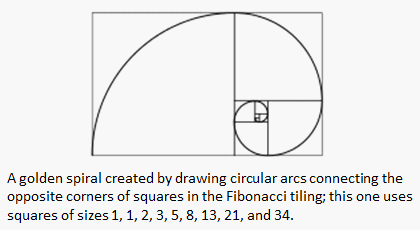# Fibonacci Numbers in MatlabThe first two Fibonacci numbers are 0 and 1, and each remaining number is the sum of the previous two. Some sources neglect the initial 0, and instead beginning the sequence with the first two ones.

The Fibonnacci numbers are also known as the Fibonacci series. Two consecutive numbers in this series are in a 'Golden Ratio'.

In mathematics and arts, two quantities are in the golden ratio if the ratio of the sum of the quantities to the larger quantity equals the ratio of the larger quantity to the smaller one.

The golden ratio is an irrational constant, approximately 1.618033988. We're designing below a simple function to see how it appears...

The command 'num2string' changes a number into a string, so that it can be included in another string. The command 'num2str(golden_ratio, 10)' shows the number 'golden_ratio' with 9 digits after the decimal point.

The simple code is here:

%Clear screen and memory
clear; clc; format compact

% Initialize the first two values
f(1) = 1;
f(2) = 1;

% Create the first 30 Fibonacci numbers
for i = 3 : 30

% Perform the sum of terms accordingly
f(i) = f(i-1) + f(i-2);

% Calculate and display the ratio of 2 consecutive elements     % of the series
golden_ratio = f(i)/f(i-1);
str = [num2str(f(i))
' ' num2str(f(i-1)) ' ' ...
num2str(golden_ratio, 10)];
disp(str)

end

Each line shows three elements: a number in the series, its predecessor and the quotient of the first number divided by the second. The results in Matlab are here:

2 1 2
3 2 1.5
5 3 1.666666667
8 5 1.6
13 8 1.625
21 13 1.615384615
34 21 1.619047619
55 34 1.617647059
89 55 1.618181818
144 89 1.617977528
233 144 1.618055556
377 233 1.618025751
610 377 1.618037135
987 610 1.618032787
1597 987 1.618034448
2584 1597 1.618033813
4181 2584 1.618034056
6765 4181 1.618033963
10946 6765 1.618033999
17711 10946 1.618033985
28657 17711 1.61803399
46368 28657 1.618033988
75025 46368 1.618033989
121393 75025 1.618033989
196418 121393 1.618033989
317811 196418 1.618033989
514229 317811 1.618033989
832040 514229 1.618033989

You can see that, in fact, the quotient of two consecutive numbers reaches the 'golden ratio' after just a few numbers in the series.

From 'Fibonacci Numbers' to home

From 'Fibonacci Numbers' to 'Matlab programming' Menu